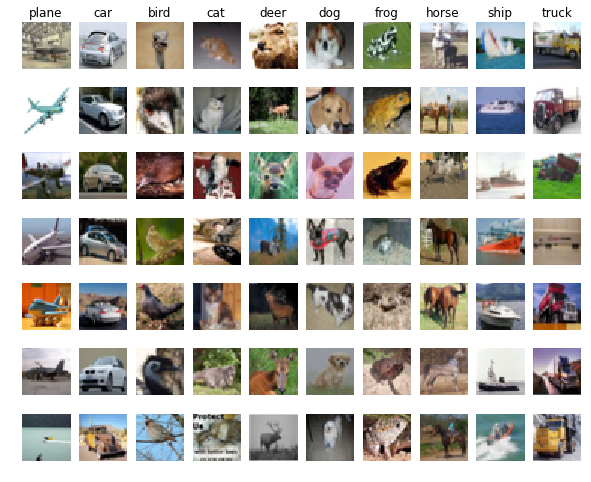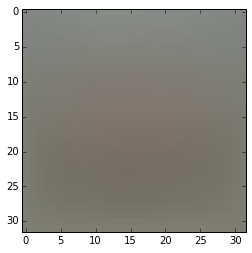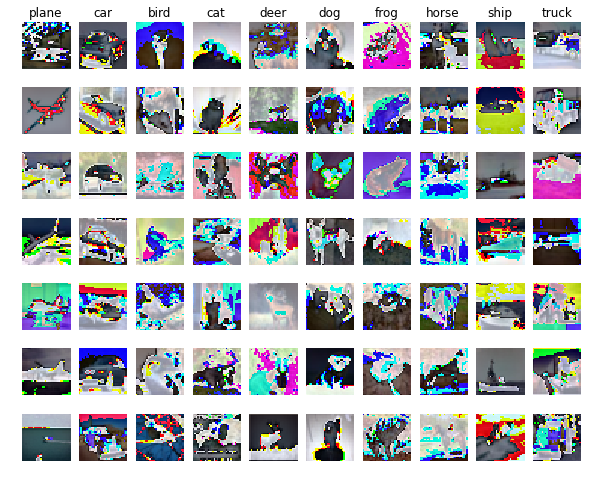In :
import random

import numpy as np
import matplotlib.pyplot as plt

# plotting setting
%matplotlib inline
plt.rcParams['figure.figsize'] = (10.0, 8.0) # set default size of plots
plt.rcParams['image.interpolation'] = 'nearest'
plt.rcParams['image.cmap'] = 'gray'

In :
# Load the raw CIFAR-10 data.
cifar10_dir = 'cs231n/datasets/cifar-10-batches-py'
X_train, y_train, X_test, y_test = load_CIFAR10(cifar10_dir)

# As a sanity check, we print out the size of the training and test data.
print('Train data shape:', X_train.shape)
print('Train labels shape:', y_train.shape)
print('Test data shape:', X_test.shape)
print('Test labels shape:', y_test.shape)

Train data shape: (50000, 32, 32, 3)
Train labels shape: (50000,)
Test data shape: (10000, 32, 32, 3)
Test labels shape: (10000,)

In :
# Visualize some examples from the dataset.
# We show a few examples of training images from each class.
classes = ['plane', 'car', 'bird', 'cat', 'deer', 'dog', 'frog', 'horse', 'ship', 'truck']
num_classes = len(classes)
samples_per_class = 7
plot_choices = []

# create random state to keep the result of randomization
rs = np.random.RandomState(seed=5566)
for y, cls in enumerate(classes):
idxs = np.flatnonzero(y_train == y)
idxs = rs.choice(idxs, samples_per_class, replace=False)
plot_choices.append(idxs)
for i, idx in enumerate(idxs):
plt_idx = i * num_classes + y + 1
plt.subplot(samples_per_class, num_classes, plt_idx)
plt.imshow(X_train[idx].astype('uint8'))
plt.axis('off')
if i == 0:
plt.title(cls)
plt.show()In :
# Subsample the data for more efficient code execution in this exercise.
num_training = 49000
num_validation = 1000
num_test = 1000

# Our validation set will be num_validation points from the original
# training set.
mask = range(num_training, num_training + num_validation)

# Our training set will be the first num_train points from the original
# training set.

# We use the first num_test points of the original test set as our
# test set.

print('Train data shape:', X_train.shape)
print('Train labels shape:', y_train.shape)
print('Validation data shape:', X_val.shape)
print('Validation labels shape:', y_val.shape)
print('Test data shape:', X_test.shape)
print('Test labels shape:', y_test.shape)

Train data shape: (49000, 32, 32, 3)
Train labels shape: (49000,)
Validation data shape: (1000, 32, 32, 3)
Validation labels shape: (1000,)
Test data shape: (1000, 32, 32, 3)
Test labels shape: (1000,)

In :
# Preprocessing: reshape the image data into rows
X_train = np.reshape(X_train, (X_train.shape, -1))
X_val = np.reshape(X_val, (X_val.shape, -1))
X_test = np.reshape(X_test, (X_test.shape, -1))

# As a sanity check, print out the shapes of the data
print('Train data shape:', X_train.shape)
print('Validation data shape:', X_val.shape)
print('Test data shape:', X_test.shape)

Train data shape: (49000, 3072)
Validation data shape: (1000, 3072)
Test data shape: (1000, 3072)

In :
# Preprocessing: subtract the mean image
# first: compute the image mean based on the training data
mean_image = np.mean(X_train, axis=0)
print(mean_image[:10])   # print a few of the elements
plt.figure(figsize=(4,4))
plt.imshow(mean_image.reshape((32,32,3)).astype('uint8'))  # visualize the mean image
plt.show()

[ 130.64189796  135.98173469  132.47391837  130.05569388  135.34804082
131.75402041  130.96055102  136.14328571  132.47636735  131.48467347]In :
# second: subtract the mean image from train and test data
X_train -= mean_image
X_val -= mean_image
X_test -= mean_image

In :
# third: append the bias dimension of ones (i.e. bias trick) so that our SVM
# only has to worry about optimizing a single weight matrix W.
# Also, lets transform both data matrices so that each image is a column.
X_train = np.hstack([X_train, np.ones((X_train.shape, 1))]).T
X_val = np.hstack([X_val, np.ones((X_val.shape, 1))]).T
X_test = np.hstack([X_test, np.ones((X_test.shape, 1))]).T

print(X_train.shape, X_val.shape, X_test.shape)

(3073, 49000) (3073, 1000) (3073, 1000)

In :
# create random state to keep the result of randomization
rs = np.random.RandomState(seed=5566)
for y, cls in enumerate(classes):
for i, idx in enumerate(plot_choices[y]):
plt_idx = i * num_classes + y + 1
plt.subplot(samples_per_class, num_classes, plt_idx)
plt.imshow(X_train[:-1, idx].astype('uint8').reshape((32, 32, 3)))
plt.axis('off')
if i == 0:
plt.title(cls)
plt.show()In [ ]: